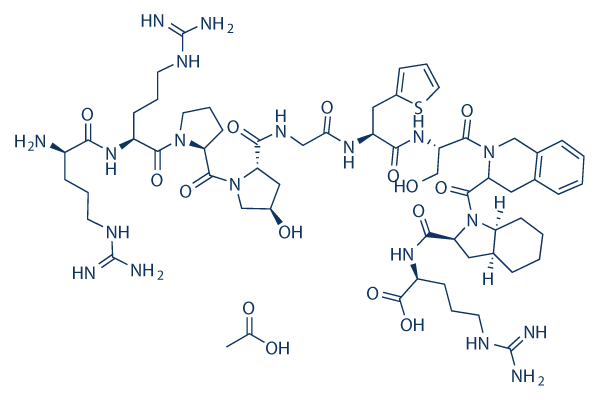# Icatibant Acetate

For research use only.

Catalog No.S5695CAS No. 138614-30-9

Icatibant Acetate acts as an antagonist of the bradykinin B2 receptor and is used in the treatment of hereditary angioedema.

### Biological Activity

Description Icatibant Acetate acts as an antagonist of the bradykinin B2 receptor and is used in the treatment of hereditary angioedema.
Targets
In vitro

Icatibant is a decapeptide resistant to the bradykinin-degrading proteases, resulting in a longer half-life than bradykinin which it antagonizes.

In vivo Icatibant exhibits efficacy in bradykinin-mediated angioedema attacks, and induces rapid symptom relief.

### Solubility (25°C)

 In vitro Water 11 mg/mL (8.06 mM) Ethanol ''-1 mg/mL

* Please note that Selleck tests the solubility of all compounds in-house, and the actual solubility may differ slightly from published values. This is normal and is due to slight batch-to-batch variations.

### Chemical Information

Molecular Weight 1364.57 C61H93N19O15S 138614-30-9 3 years-20°C powder 2 years-80°C in solvent N/A C1CCC2C(C1)CC(N2C(=O)C3CC4=CC=CC=C4CN3C(=O)C(CO)NC(=O)C(CC5=CC=CS5)NC(=O)CNC(=O)C6CC(CN6C(=O)C7CCCN7C(=O)C(CCCN=C(N)N)NC(=O)C(CCCN=C(N)N)N)O)C(=O)NC(CCCN=C(N)N)C(=O)O

### In vivo Formulation Calculator (Clear solution)

 Step 1: Enter information below (Recommended: An additional animal making an allowance for loss during the experiment) Dosage mg/kgAverage weight of animals gDosing volume per animal ulNumber of animals Step 2: Enter the in vivo formulation (This is only the calculator, not formulation. Please contact us first if there is no in vivo formulation at the solubility Section.) % DMSO+ % PEG300 Corn oil + % Tween 80+ % ddH2O CalculateReset

### Bio Calculators

Molarity Calculator

## Molarity Calculator

Calculate the mass, volume or concentration required for a solution. The Selleck molarity calculator is based on the following equation:

### Mass (mg) = Concentration (mM) × Volume (mL) × Molecular Weight (g/mol)

• Mass
Concentration
Volume
Molecular Weight

*When preparing stock solutions, please always use the batch-specific molecular weight of the product found on the via label and SDS / COA (available on product pages).

Dilution Calculator

## Dilution Calculator

Calculate the dilution required to prepare a stock solution. The Selleck dilution calculator is based on the following equation:

### Concentration (start) x Volume (start) = Concentration (final) x Volume (final)

This equation is commonly abbreviated as: C1V1 = C2V2 ( Input Output )

• C1
V1
C2
V2

* When preparing stock solutions always use the batch-specific molecular weight of the product found on the vial label and SDS / COA (available online).

## The Serial Dilution Calculator Equation

• ### Computed Result

• C1=C0/X C2=C1/X C1: LOG(C1): C2: LOG(C2): C3: LOG(C3): C4: LOG(C4): C5: LOG(C5): C6: LOG(C6): C7: LOG(C7): C8: LOG(C8):
Molecular Weight Calculator

## Molecular Weight Calculator

Enter the chemical formula of a compound to calculate its molar mass and elemental composition:

### Total Molecular Weight: g/mol

Tip: Chemical formula is case sensitive. C10H16N2O2 c10h16n2o2

#### Instructions to calculate molar mass (molecular weight) of a chemical compound:

To calculate molar mass of a chemical compound, please enter its chemical formula and click 'Calculate'.

#### Definitions of molecular mass, molecular weight, molar mass and molar weight:

Molecular mass (molecular weight) is the mass of one molecule of a substance and is expressed in the unified atomic mass units (u). (1 u is equal to 1/12 the mass of one atom of carbon-12)
Molar mass (molar weight) is the mass of one mole of a substance and is expressed in g/mol.

#### Molarity Calculator

 Mass Concentration Volume Molecular Weight pg ng μg mg g kg = fM pM nM μM mM M × nL μL mL L ×

### Tech Support

Answers to questions you may have can be found in the inhibitor handling instructions. Topics include how to prepare stock solutions, how to store inhibitors, and issues that need special attention for cell-based assays and animal experiments.

Tel: +1-832-582-8158 Ext:3